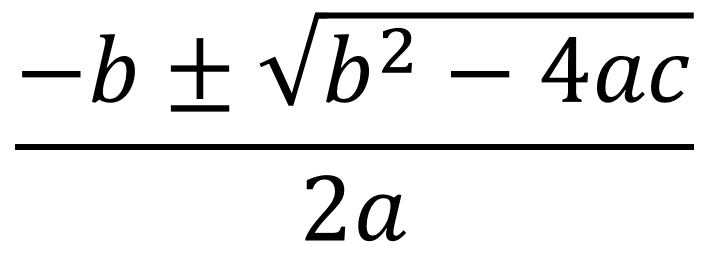Home > High School: Algebra > Quadratic Formula

Directions: Using the digits 1 to 9, at most one time each for a, b, and c, find the result with the greatest and least value:Hint

What will the exponent do?

Assuming the solution can be negative, the greatest value would occur when a=2, b=9, and c=1 (solution = -0.11). The least value, found by Robert Hays, would occur when a=1, b=9, c=2 (solution = -8.77)

Source: Dane Ehlert

Writing Equivalent Polynomial Expressions

Directions: Use the digits 1-9, at most one time each, to create a true statement. …

1.I agree with the largest value a=2, b=9, c=1 ( solution = 0.11 )

The smallest value I found was a=1, b=9, c=2 ( solution = -8.77 )

•Thanks Robert! The answer has been updated.

•a=1, b=9, c= 1 gives -0.11251…
a=2, b=9, c=1 gives -0.113999…

•Robert Kaplinsky

Thanks MRO. The problem with a=1, b=9, c= 1 is that you cannot repeat a digit.